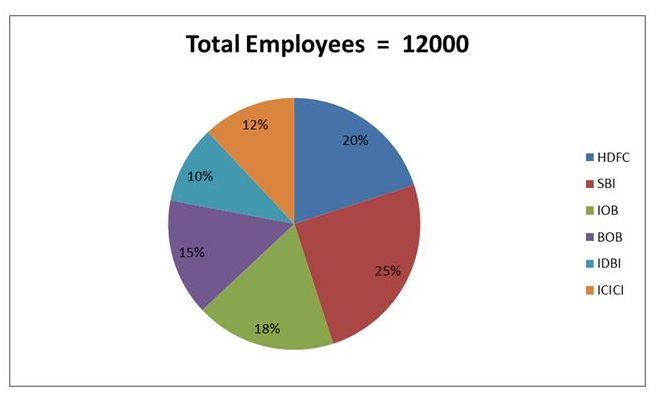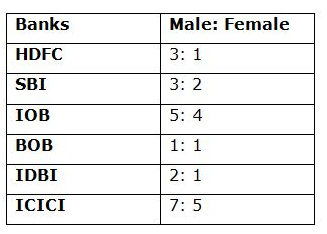# RRB Clerk Prelims Quantitative Aptitude (Day-13)

## Quantitative Aptitude Materials for RRB Clerk

Dear Aspirants, Our IBPS Guide team is providing new series of Quantitative Aptitude Questions for RRB Clerk 2020 Prelims so the aspirants can practice it on a daily basis. These questions are framed by our skilled experts after understanding your needs thoroughly. Aspirants can practice these new series questions daily to familiarize with the exact exam pattern and make your preparation effective.

Start Quiz

Ensure Your Ability Before the Exam Take IBPS RRB Clerk 2020 Prelims Free Mock Test

Data Interpretation

Direction (1-6): Study the following information carefully and answer the questions given below.

The given pie chart shows the number of employee works in different banks – HDFC, SBI, IOB, BOB, IDBI and ICICI in 2019.The given table shows the ratio of the number of male to female employees in different banks.1) The number of female employees in IDBI is approximately what percent of the total number of employees in ICICI?

A) 22%

B) 26%

C) 28%

D) 30%

E) 32%

2) What is the difference between the average number of male employees from HDFC, BOB and ICICI together and the average number of female employees from SBI and IOB together?

A) 80

B) 100

C) 110

D) 120

E) None of these

3) What is the ratio of the number of female employees from HDFC and ICICI together to the number of male employees from IOB and IDBI together?

A) 1: 3

B) 3: 5

C) 5: 7

D) 4: 7

E) None of these

4) What is the average number of female employees from all the banks excluding ICICI?

A) 808

B) 810

C) 812

D) 814

E) None of these

5) Number of male employees from IDBI is approximately what percent of the total number of employees from IOB?

A) 37%

B) 39%

C) 41%

D) 43%

E) 33%

6) What is the difference between the total number of employees from HDFC and the number of male employees from SBI?

A) 600

B) 400

C) 300

D) 500

E) None of these

Approximation

Direction (7-8): What approximate value should come in the place of (?) in the following questions?

7) (319.897 ÷ √65) + 50.12 % of 119.92 =?

A) 90

B) 80

C) 50

D) 70

E) 100

8) 8.912 * 6.076 + 65.102 % of 39.912 + 5.902 * 2.101 =?

A) 88

B) 92

C) 96

D) 84

E) 100

Direction (9-10): Following question contains two equations as I and II. You have to solve both equations and determine the relationship between them and give answer as,

a) If x > y

b) If x ≥ y

c) If x = y or relationship cannot be determined.

d) If x < y

e) If x ≤ y

9)

I) x2+ 24x + 119 = 0

II) y2+ 13y + 42 = 0

10)

I) x2– 19x + 88 = 0

II) y2– 21y + 104 = 0

Directions (1-6) :Required percentage = 400/1440 * 100 = 28%

Average male employees from HDFC, BOB and ICICI = (1800 + 900 + 840)/3

= 1180

Average female employee from SBI and IOB = (1200 + 960)/2 = 1080

Difference = 1180 – 1080 = 100

Required ratio = (600 + 600): (1200 + 800)

= 1200: 2000

= 3: 5

Required average = (600 + 1200 + 960 + 900 + 400)/5

= 812

Required percentage = 800/2160 * 100

= 37%

Difference = 2400 – 1800 = 600

Directions (7-8) :

(319.897 ÷ √65) + 50.12 % of 119.92 =?

320/8 + 1/2 * 120 =?

? = 100

8.912 * 6.076 + 65.102 % of 39.912 + 5.902 * 2.101 =?

54 + 26 + 12 =?

92 =?

Directions (9-10):

x2 + 24x + 119 = 0

x2 + 17x + 7x + 119 = 0

x(x + 17) + 7(x + 17) = 0

(x + 7)(x + 17) = 0

x = -7, -17

y2 + 13y + 42 = 0

y2 + 6y + 7y + 42 = 0

y(y + 6) + 7(y + 6) = 0

(y + 7)(y + 6) = 0

Y = -7, -6

Hence, x ≤ y

x2 – 19x + 88 = 0

x2 – 11x – 8x + 88 = 0

x(x – 11) – 8(x – 11) = 0

(x – 8)(x – 11) = 0

x = 8, 11

y2 – 21y + 104 = 0

y2 – 8y – 13y + 104 = 0

y(y – 8) – 13(y – 8) = 0

(y – 13)(y – 8) = 0

y = 13, 8

Relationship between x and y cannot be established.

 Check Here to View RRB Clerk Prelims 2020 Quantitative Aptitude Questions Day – 12 Day – 11 Day – 10
5 1 vote
Rating